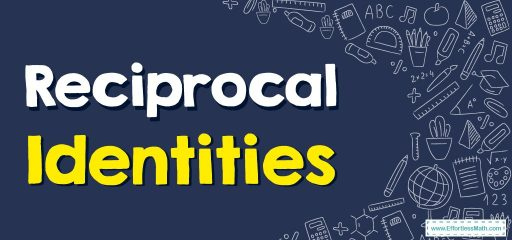# Reciprocal Identities

Every fundamental trigonometric function is the reciprocal of other trigonometric functions. In this step-by-step guide, you will learn more about reciprocal identities.## A step-by-step guide toreciprocal identities

The reciprocals of the six basic trigonometric functions ($$sin$$, $$cos$$, $$tan$$, $$sec$$, $$csc$$, $$cot$$) are called reciprocal identities. Reciprocal identities are important trigonometric identities that are used to solve various problems in trigonometry.

The $$sin$$ function is the reciprocal of the $$csc$$ function and vice-versa; the $$cos$$ function is the reciprocal of the $$sec$$ function and vice-versa; the $$cot$$ function is the reciprocal of the $$tan$$ function and vice-versa.

The formulas of the six main reciprocal identities are:

• $$\color{blue}{sin\:\left(\theta \right)=\frac{1}{csc\:\left(\theta \right)}}$$
• $$\color{blue}{cos\:\left(\theta \right)=\frac{1}{sec\:\left(\theta \right)}}$$
• $$\color{blue}{tan\:\left(\theta \right)=\frac{1}{cot\:\left(\theta \right)}}$$
• $$\color{blue}{csc\:\left(\theta \right)=\frac{1}{sin\:\left(\theta \right)}}$$
• $$\color{blue}{sec\:\left(\theta \right)=\frac{1}{cos\:\left(\theta \right)}}$$
• $$\color{blue}{cot\:\left(\theta \right)=\frac{1}{tan\:\left(\theta \right)}}$$

### Reciprocal Identities – Example 1:

Find the value of $$sec\: x$$ if $$cos\: x = \frac{2}{9}$$ using the reciprocal identity.

Solution

We know the reciprocal identity $$sec\: x = \frac{1}{cos x}$$

So, if $$cos\: x = \frac{2}{9}$$, then:

$$sec\:x=\:\frac{1}{cos\:x}=\frac{1}{\frac{2}{9}}=\frac{9}{2}$$

### What people say about "Reciprocal Identities - Effortless Math: We Help Students Learn to LOVE Mathematics"?

No one replied yet.

X
30% OFF

Limited time only!

Save Over 30%

SAVE $5 It was$16.99 now it is \$11.99